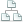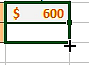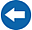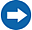Home >Tips >Excel 2016 from Scratch >Calculate sums, max, average, medianSite map

# An Excel tutorial by Peter KalmstromIn this demo in the Excel 2016 from Scratch series, Peter Kalmstrom shows the solution to an exercise where sums need to be calculated along with, maximum, average and median values of selected cells.

As an example, Peter uses calls from different departments going through a switchboard during the hours of the day.

### Exercise

If you want to try the exercise yourself, please download the Excel file Peter uses in the demo.

### Content

This is what Peter shows in the demo below:
• How to use an empty column to separate number cells that should be included in a calculation from number cells that should not be included.
•How to use the fill to enter the same kind of values and formatting in more cells.
• How to create a formula that finds the maximum value in the selected cells.
• How to create a formula that calculates the average value of the values in the selected cells.
• How to create a formula that finds the median value of the values in the selected cells.
• How to use Cell Styles to differentiate between input cells and calculation cells with formulas.
• How to change the number of decimals shown in all cells.

Peter uses Excel 2016 for his demo, but the Excel basics are the same for earlier versions of Excel.